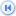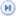Spike's Calculators

# Timber/Lumber Volume Units

The units that are(were) used for the timber/lumber products volume conversions.

Throughout Europe lumber cargoes where typically measured in standards (standard hundred). These standards differed by location but in general they consisted of 120 pieces adding up to a certain amount in board feet and cubic feet. They were used for shipping, buying and selling of lumber products.

In addition to these there are terms used to measure fuel wood, wood chips and pulp.

##### Note:
The abbreviations used with the units are abbreviations we use on this Web site. For instance, the Petrograd Standard, in some of the publications is this term is abbreviated as P.S.H = Petrograd Standard Hundred.
The board foot (American) and superficial foot (English) both the same in size.

Unit NameConversion typeSystem type/ used in Note Imperial Metric
American standard (Am.std)Timber/Lumber VolumeTimber/Lumber trade120 pieces - 2" x 12" x 12' = 1237½ bf 1 American standard = 240 cubic feet (ft³)1 American standard = 6.796 cubic metres (m³)
Blodgett foot (Bl ft)Timber/Lumber VolumeNorth Americalog rule that was used in the Northeastern United States1 Blodgett foot (Bl ft) = 1.396 cubic feet (ft³)1 Blodgett foot (Bl ft) = 0.0396 cubic metres (m³)
board foot (bf)Timber/Lumber VolumeNorth America1 bf = 12" x 12" x 1" = 144 cubic inches1 board foot (bf) = 0.0833 cubic feet (ft³)1 board foot (bf) = 0.0024 cubic metres (m³)
carload (C/L)Timber/Lumber VolumeNorth America15000 superficial feet B.M.of 1 inch . American hardwood lumber contract definition. carload (C/L) = rail car1 carload = 1250 cubic feet (ft³)1 carload = 35.394 cubic metres (m³)
centistere (cst)Volumemetric unit (old)100 cst = one stere1 centistere (cst) = 0.353 cubic feet (ft³)1 centistere (cst) = 0.01 cubic metres (m³)
Christiana standard (Cri.std)Timber/Lumber VolumeTimber/Lumber trade120 pieces - 1¼" x 9" x 11' = 1237½ bf 1 Christiana standard (Cri.std) = 103.125 cubic feet (ft³)1 Christiana standard (Cri.std) = 2.92 cubic metres (m³)
cord (cd) Timber/Lumber VolumeNorth Americastack of firewood 4 ft wide, 8 ft long, and 4 ft high1 cord (cd) = 128 cubic feet (ft³)1 cord (cd) = 3.625 cubic metres (m³)
cord footTimber/Lumber VolumeNorth Americastack of firewood 4 ft wide x 1 ft long, 4 ft high = 1/8 of a cord1 cord foot (cd ft) = 16 cubic feet (ft³)1 cord foot (cd ft) = 0.453 cubic metres (m³)
cubic foot (ft³) VolumeImperial unitBasic Imperial volume unit used for timber volume1 cubic foot (ft³) = 1 cubic feet (ft³)1 cubic foot (ft³) = 0.0283 cubic metres (m³)
cubic metre (m³)VolumeMetric unitcubic metre is a SI base unit1 cubic metre (m³) = 35.315 cubic feet (ft³)1 cubic metre (m³) = 1 cubic metres (m³)
cubic shaku (shaku³) VolumeJapan1 cubic shaku = 12 boardfeet Brereton log scale1 cubic shaku (shaku³) = 0.9826 cubic feet (ft³)1 cubic shaku (shaku³) = 0.0278 cubic metres (m³)
cubic ton (t³) Timber/Lumber VolumeImperial unit Also known as timber ton or measurement ton1 cubic ton (t³) = 40 cubic feet (ft³)1 cubic ton (t³) = 1.132 cubic metres (m³)
cunit (cunit)Timber/Lumber VolumeCanadianUsed for wood intended as pulpwood or firewood. 100 ft³ solid wood, and also used for 100 ft³ of sawdust or 100 ft³ pulp chips1 cunit (cunit) = 100 cubic feet (ft³)1 cunit (cunit) = 2.83 cubic metres (m³)
Dantzig standardsTimber/Lumber VolumeTimber/Lumber trade120 pieces, 1½" x 12" x 12'1 Dantzig standard (Dan.std) = 180 cubic feet (ft³)1 Dantzig standard (Dan.std) = 5.097 cubic metres (m³)
decastere (dast)Volumemetric unit (old)1 dast = 10 st1 decastere (dast) = 353.147 cubic feet (ft³)1 decastere (dast) = 10 cubic metres (m³)
decistere (dst)Volumemetric unit (old)10 dst = 1 stere1 decistere (dst) = 3.53 cubic feet (ft³)1 decistere (dst) = 0.1 cubic metres (m³)
Drammen standard (Drm.std)Timber/Lumber VolumeTimber/Lumber trade120 pieces - 2½" x 6½" x 12' = 1462½ bf1 Drammen standard (Drm.std) = 121.875 cubic feet (ft³)1 Drammen standard (Drm.std) = 3.45 cubic metres (m³)
Drontheim standard (round timber)Timber/Lumber VolumeTimber/Lumber trade1728 board feet (bf)1 Drontheim standard (round timber) = 144 cubic feet (ft³)1 Drontheim standard (round timber) = 4.078 cubic metres (m³)
Drontheim standard (sawn deals)Timber/Lumber VolumeTimber/Lumber trade2376 board feet (bf)1 Drontheim standard (sawn deals) = 198 cubic feet (ft³)1 Drontheim standard (sawn deals) = 5.6 cubic metres (m³)
Drontheim standard (square timber)Timber/Lumber VolumeTimber/Lumber trade2160 board feet (bf)1 Drontheim standard (square timber) = 180 cubic feet (ft³)1 Drontheim standard (square timber) = 5.097 cubic metres (m³)
face cord (f.cd)Timber/Lumber VolumeNorth Americastack of firewood 16 in wide, 8 ft long, and 4 ft high. We use a value of 16 inches for the width, but the width can be anything from 12" to 36"1 face cord (f cd) = 42.667 cubic feet (ft³)1 face cord (f cd) = 1.208 cubic metres (m³)
fathom (pftm)Timber/Lumber VolumeImperial unit1 fathom = 6 feet. A piled fathom = 6' x 6' x 6'1 fathom (pftm) = 216 cubic feet (ft³)1 fathom (pftm) = 6.116 cubic metres (m³)
fathom (pftm) RussianTimber/Lumber VolumeRussian unit1 Russian fathom = 7 feet. A piled Russian fathom = 7' x 7' x7'1 Russian fathom (Rus.pftm) = 343 cubic feet (ft³)1 Russian fathom (Rus.pftm) = 9.71cubic metres (m³)
Francon cubic metre (fr.m³)Timber/Lumber VolumeImperial unitHoppus using metric units1 Francon cubic metre (fr.m³) = 44.964 cubic feet (ft³) 1 Francon cubic metre (fr.m³) = 1.273 cubic metres (m³)
French cord (cd) FrenchTimber/Lumber VolumeCanada, Quebec8 French feet long, 4 French feet high and 3 English feet wide. A french foot = 12.789 inches1 French cord (cd) French = 109.039014 cubic feet (ft³)1 French cord (cd) French = 4.0925 cubic metres (m³)
Gefle standard (Gef.std)Timber/Lumber VolumeTimber/Lumber tradeUsed for telegraph post1 Gefle standard (Gef.std) = 100 cubic feet (ft³)1 Gefle standard (Gef.std) = 2.8 cubic metres (m³)
Gothenburg standard (Got.std)Timber/Lumber VolumeTimber/Lumber tradeUsed for pit props, pile of round wood 6 ft x 6 ft x 5 ft = 180 ft³ or as sawn lumber 1 Gothenburg standard (Got.std) = 180 cubic feet (ft³)1 Gothenburg standard (Got.std) = 5.097 cubic metres (m³)
Haakondahl superficial foot (Hdf)Timber/Lumber Volume New Zealandused in New Zealand. Same as Hoppus board foot1 Haakondahl superficial foot (Hdf) = 0.106 cubic feet (ft³)1 Haakondahl superficial foot (Hdf) = 0.003 cubic metres (m³)
hectostere (hst)Volumemetric unit (old)1 hectostre = 100 steres1 hectostere (hst) = 3531.47 cubic feet (ft³)1 hectostere (hst) = 100 cubic metres (m³)
Hoppus foot (h ft)Timber/Lumber VolumeImperial unitquarter girth formula1 Hoppus foot (h ft)1 = 0.273 cubic feet (ft³) 1 Hoppus foot (h ft) = 0.0360541289 cubic metres (m³)
Hoppus superficial foot (hs ft)Timber/Lumber VolumeImperial unitHoppus foot = 12 Hoppus superficial foot 1 Hoppus superficial foot (hs ft) = 0.106 cubic feet (ft³)1 Hoppus superficial foot (hs ft) = 0.003 cubic metres (m³)
Hoppus ton (h tn)Timber/Lumber VolumeImperial unitShipments of tropical hardwoods from Southeast Asia, still used in Burma1 Hoppus ton (h tn) = 63.66 cubic feet (ft³)1 Hoppus ton (h tn) = 1.8 cubic metres (m³)
kilostere (kst)Volumemetric unit (old)1 kilosteres = 1000 steres1 kilostere (kst) = 35314.7 cubic feet (ft³)1 kilostere (kst) = 1000 cubic metres (m³)
kokuVolume Japan1 koku = 120 board feet, Breteon log scale1 koku = 9.826 cubic feet (ft³)1 koku = 0.278 cubic metres (m³)
lathwoodTimber/Lumber VolumeTimber/Lumber trade1 cubic fathom (pftm) lathwood contains about 180 - 200 pieces = 288 cubic feet1 Lathwood = 288 cubic feet (ft³)1 Lathwood = 8.155 cubic metres (m³)
London standard (Lon.std)Timber/Lumber VolumeTimber/Lumber tradeIrish/London standard. 120 pieces - 3" x 9" x 12' = 3240 bf1 London standard (Lon.std) = 270 cubic feet (ft³)1 London standard (Lon.std) = 7.646 cubic metres (m³)
million board feet (mmbf)Timber/Lumber VolumeNorth AmericaMmbf = 1000000 board feet1 million board foot (mmbf) = 83333.3 cubic feet (ft³)1 million board foot (mmbf) = 2359.74 cubic metres (m³)
millistere (mst)Volumemetric unit (old)one millistere is equivalent to one litre1 millistere (mst) = 0.0353147 cubic feet (ft³)1 millistere (mst) = 0.001 cubic metres (m³)
Norway standardsTimber/Lumber VolumeTimber/Lumber trade120 pieces, 3" x 9" x 12'1 Norway standard (Nor.std) = 270 cubic feet (ft³)1 Norway standard (Nor.std) = 7.646 cubic metres (m³)
Petrograd standard (Ptg.std)Timber/Lumber VolumeTimber/Lumber tradePetrograd Standard Hundred (PSH). 120 pieces - 1½" x 11" x 12' = 1980 bf1 Petrograd standard (Ptg.std) = 165 cubic feet (ft³). 1 Petrograd standard (Ptg.std) = 4.672 cubic metres (m³)
Quebec standard (Que.std)Timber/Lumber VolumeTimber/Lumber trade100 pieces - 2½" x 11" x 12' = 2750 bf1 Quebec standard (Que.std) = 229.167 cubic feet (ft³)1 Quebec standard (Que.std) = 6.489 cubic metres (m³)
Riga last (Rl round timber)Timber/Lumber VolumeBritish timber measureLast is an old North European measurement used for measuring large quantities.1 Riga last (Rl round timber) = 65 cubic feet (ft³)1 Riga last (Rl round timber) = 1.841 cubic metres (m³)
Riga last (Rl square timber)Timber/Lumber VolumeBritish timber measure Last is a German word for load. 1 Riga last (Rl square timber) = 80 cubic feet (ft³)1 Riga last (Rl square timber) = 2.265 cubic metres (m³)
rullyTimber/Lumber VolumeDanube lumber measurement12 ft x 6 ft x 3ft = one fathom1 rully = 216 cubic feet (ft³)1 rully = 6.116 cubic metres (m³)
stack of woodTimber/Lumber VolumeNorth Americaold English measure of fuel wood. Cut firewood 1 stack = 4 cubic yards1 stack of wood = 108 cubic feet (ft³)1 stack of wood = 3.058 cubic metres (m³)
stere (st)Timber/Lumber Volumemetric unit (old)used to measure stacked timber/firewood1 stere (st) = 35.3147 cubic feet (ft³)1 stere (st) = 1 cubic metres (m³)
Sweden standardsTimber/Lumber VolumeTimber/Lumber trade120 pieces, 3" x 9" x 14'1 Sweden standard (Swe.std) = 315 cubic feet (ft³)1 Sweden standard (Swe.std) = 8.92 cubic metres (m³)
thousand board feet (mbf)Timber/Lumber VolumeNorth Americambf = 1000 * 1 bf1 thousand board foot (mbf) = 83.333 cubic feet (ft³)1 thousand board foot (mbf) = 2.36 cubic metres (m³)
Upper Gulf hewn balksTimber/Lumber VolumeTimber/Lumber trade a roughly hewn piece of timber.1 Upper Gulf hewn balks = 150 cubic feet (ft³)1 Upper Gulf hewn balks = 4.25 cubic metres (m³)
volumetric unit (vu)Timber/Lumber VolumeNorth Americameasurement used for woodchips equal to a volume of 200 cubic feet 1 volumetric unit (vu) = 200 cubic feet (ft³)1 volumetric unit (vu) = 5.66 cubic metres (m³)
wagon loadTimber/Lumber VolumeDanube lumber measurement1 wagon load = 22 cubic metres with an approximate weight of 10 tons1 wagon load = 776.9 cubic feet (ft³)1 wagon load = 22 cubic metres (m³)
Wyburg standard (square timber)Timber/Lumber VolumeTimber/Lumber trade1963 board feet (bf)1 Wyburg standard (square timber) = 163.667 cubic feet (ft³)1 Wyburg standard (square timber) = 4.635 cubic metres (m³)
Wyburg standard (round timber)Timber/Lumber VolumeTimber/Lumber trade1560 board feet (bf)1 Wyburg standard (round timber) = 130 cubic feet (ft³)1 Wyburg standard (round timber) = 3.68 cubic metres (m³)
Wyburg standard (sawn deals)Timber/Lumber VolumeTimber/Lumber trade2160 board feet (bf)1 Wyburg standard (sawn deals) = 180 cubic feet (ft³)1 Wyburg standard (sawn deals) = 5.097 cubic metres (m³)
StandardTimber/Lumber VolumeTimber/Lumber tradeUnit of volume used for the measurement of timber/lumber.1 standard (std) = 1980 board feet (bf) 1 Standard (std) = 165 cubic feet (ft³)1 Standard = 4.67 3.68 cubic metres (m³)